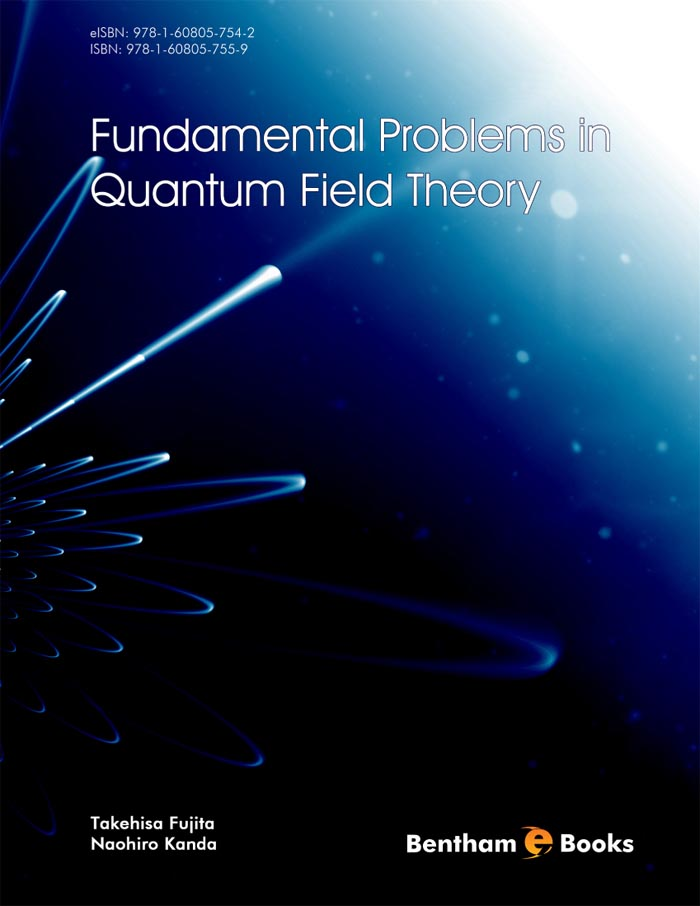### Maxwell and Dirac Equations

#### Abstract

This chapter discusses the basic equations in quantum field theory. First, we clarify some important properties of Maxwell equation so that the main part of the electromagnetisms can be easily understood. Then, we present some useful properties of the Dirac equation and its free wave solution. These two equations are the basic ingredients in understanding quantum field theory. We also give the exact energy spectrum of Dirac equation with Coulomb plus gravity potential in hydrogenlike atom

Total Pages: 3-19 (17)